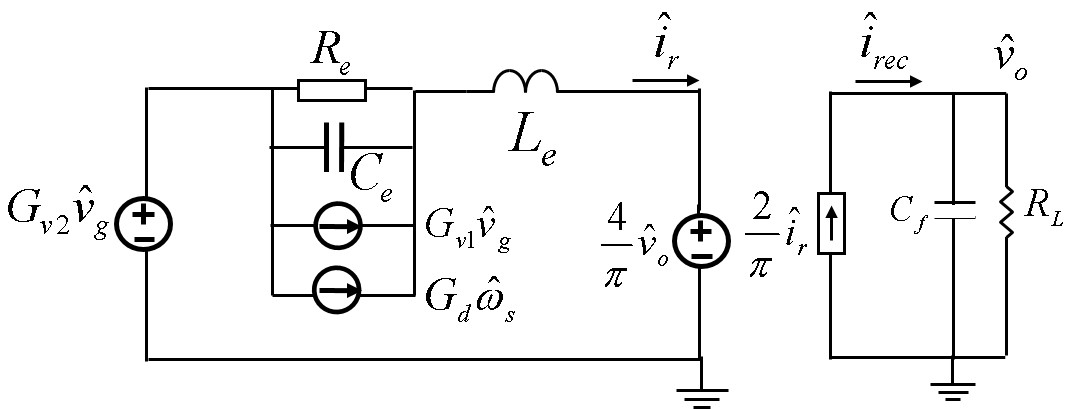LIBRARY

# Simple Equivalent Circuit Model of a Series Resonant Converter

Year: 2015Fig. 1. Schematic of full-bridge series resonant converter (SRC)
The series resonant converter (SRC), shown in Fig. 1, is the simplest resonant converter. It is widely used for power conditioning in the sophisticated aerospace industry, as well as in some industrial applications, such as laser power supply. Up to now, the most successful equivalent circuit model proposed by E. Yang was based on the extended describing function method. However, the original equivalent circuit model is a complicated fifth-order circuit, and a numerical solution is used to obtain small-signal characteristics.

This nugget simplifies the original equivalent circuit model, and proposes a simple equivalent circuit model of a series resonant converter. Shown in Fig. 2, the proposed equivalent circuit model is a third-order circuit. The components Ce and Le forms a beat frequency double pole and Re is responsible for the damping. When switching frequency fs is far away from resonant frequency fo, Re is large. The quality factor of the beat frequency double pole is large, thereby enabling its observation. In this case, the circuit is a third order with a beat frequency double pole and a low frequency single pole caused by the output filter. When switching frequency fs is close to resonant frequency fo, Re is small causing the beat frequency double pole to split. In this case, no beat frequency double pole is observed. Instead, Le will resonant with output capacitor Cf to form a low frequency double pole. With the analytical model, the beat frequency dynamics can be explained, and the region where the beat frequency double pole is observed can be determined analytically. Furthermore, for the first time, analytical expressions of the transfer functions are derived. The equivalent circuit model and analytical transfer functions are valuable tools to design the feedback control appropriately.Fig. 2. Proposed simplified equivalent circuit model